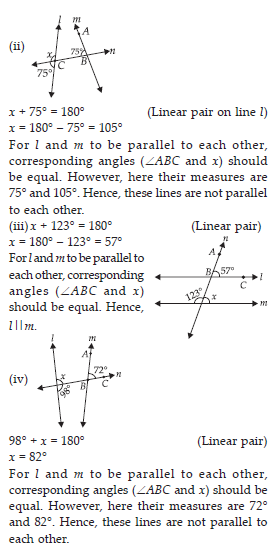# Lines and Angles (Maths) Class 7 - NCERT Questions

Q 1.

Find the complement of each of the following angles :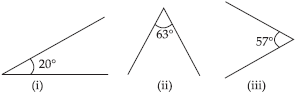SOLUTION:

The sum of the measure of complementary angles is 90°.
(i) Complement of 20° = 90° – 20° = 70°
(ii) Complement of 63° = 90° – 63° = 27°
(iii) Complement of 57° = 90° – 57° = 33°

Q 2.

Find the supplement of each of the following angles: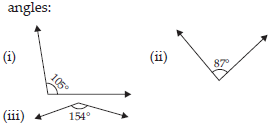SOLUTION:

The sum of measure of supplementary angles is 180°.
(i) Supplement of 105° = 180° – 105° = 75°
(ii) Supplement of 87° = 180° – 87° = 93°
(iii) Supplement of 154° = 180° – 154° = 26°

Q 3.

Identify which of the following pairs of angles are complementary and which are supplementary.
(i) 65°, 115°
(ii) 63°, 27°
(iii) 112°, 68°
(iv) 130°, 50°
(v) 45°, 45°
(vi) 80°, 10°

SOLUTION:

The sum of the measure of complementary angles is 90° and that of supplementary angles is 180°.
(i) Sum of the measure of angles = 65° + 115° = 180°
∴ These angles are supplementary angles.
(ii) Sum of the measure of angles = 63° + 27° = 90°
∴ These angles are complementary angles.
(iii) Sum of the measure of angles = 112° + 68° = 180°
∴ These angles are supplementary angles.
(iv) Sum of the measure of angles = 130° + 50° = 180°
∴ These angles are supplementary angles.
(v) Sum of the measure of angles = 45° + 45° = 90°
∴ These angles are complementary angles.
(vi) Sum of the measure of angles = 80° + 10° = 90°
∴ These angles are complementary angles.

Q 4.

Find the angle which is equal to its complement.

SOLUTION: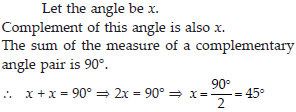Q 5.

Find the angle which is equal to its supplement.

SOLUTION:

Let the angle be x.
Supplement of this angle is also x.
The sum of the measure of a supplementary angle pair is 180°.
∴ x + x = 180° ⇒ 2x = 180° ⇒ x = 90°

Q 6.

In the given figure, ∠1 and ∠2 are supplementary angles. If ∠1 is decreased, what changes should take place in ∠2 so that both the angles still remain supplementary.

SOLUTION:

∠1 and ∠2 are supplementary angles.
If ∠1 is reduced, then ∠2 should be increased by the same measure so that this angle pair remains supplementary.

Q 7.

Can two angles be supplementary if both of them are
(i) Acute?
(ii) Obtuse?
(iii) Right?

SOLUTION:

(i) No. Acute angle is always less than 90°. It can be observed that two angles, even of 89°,
cannot add up to 180°. Therefore, two acute angles cannot form a supplementary.
(ii) No. Obtuse angle is always greater than 90°.
It can be observed that two angles, even of 91°, will always add up to more than 180°. Therefore, two obtuse angles cannot form a supplementary.
(iii) Yes. Right angles are of 90° and 90° + 90° = 180°.
Therefore, two right angles form a supplementary angle together.

Q 8.

An angle is greater than 45°. Is its complementary angle greater than 45° or equal to 45° or less than 45°?

SOLUTION:

Let A and B are two angles making a complementary angle pair and A is greater than 45°.
A + B = 90°
B = 90° – A
Therefore, B will be less than 45°.

Q 9.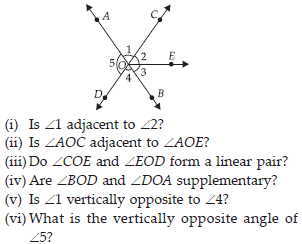SOLUTION:

(i) Yes. Since they have a common vertex O and also a common arm OC. Also, their non-common arms, OA and OE, are on the opposite side of the common arm.
(ii) No. They have a common vertex O and also a common arm OA. But, their non-common arms, OC and OE, are on the same side of the common arm. Therefore, these are not adjacent to each other.
(iii) Yes. Since they have a common vertex O and a common arm OE. Also, their non-common arms, OC and OD, are opposite rays.
(iv) Yes. Since ∠BOD and ∠DOA have a common vertex O and their non-common arms are opposite to each other.
(v) Yes. Since these are formed due to the intersection of two straight lines (AB and CD).
(vi) ∠COB is the vertically opposite angle of ∠5 as these are formed due to the intersection of two straight lines, AB and CD.

Q 10.

Indicate which pairs of angles are :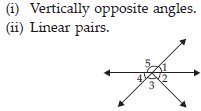SOLUTION:

(i) ∠1 and ∠4, ∠5 and ∠2 + ∠3 are vertically opposite angles as these are formed due to the intersection of two straight lines.
(ii) ∠1 and ∠5, ∠5 and ∠4 are forming linear pair as these have a common vertex and also have non-common arms opposite to each other.

Q 11.

In the following figure, is ∠1 adjacent to ∠2? Give reasons.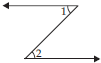SOLUTION:

∠1 and ∠2 are not adjacent angles because their vertex is not common.

Q 12.

Find the value of the angles x, y and z in each of the following: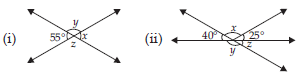SOLUTION: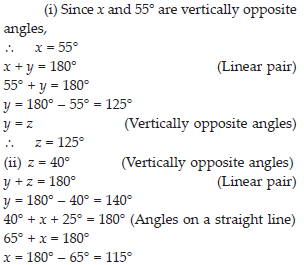Q 13.

Fill in the blanks:
(i) If two angles are complementary, then the sum of their measure is ______ .
(ii) If two angles are supplementary, then the sum of their measure is ______ .
(iii) Two angles forming a linear pair are ______ .
(iv) If two adjacent angles are supplementary, they form a _____ .
(v) If two lines intersect at a point, then the vertically opposite angles are always ____ .
(vi) If two lines intersect at a point, and if one pair of vertically opposite angles are acute angles, then the other pair of vertically opposite angles are _____ .

SOLUTION:

(i) 90°
(ii) 180°
(iii) supplementary
(iv) linear pair
(v) equal
(vi) obtuse angles

Q 14.

In the adjoining figure, name the following pairs of angles.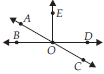(i) Obtuse vertically opposite angles
(iii) Equal supplementary angles
(iv) Unequal supplementary angles
(v) Adjacent angles that do not form a linear pair

SOLUTION:

(i) ∠AOD, ∠BOC
(ii) ∠EOA, ∠AOB
(iii) ∠EOB, ∠EOD
(iv) ∠EOA, ∠EOC
(v) ∠AOB and ∠AOE; ∠AOE and ∠EOD; ∠EOD and ∠DOC

Q 15.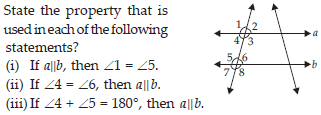SOLUTION:

(i) Corresponding angles property
(ii) Alternate interior angles property
(iii) Interior angles on the same side of transversal are supplementary.

Q 16.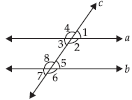(i) the pairs of corresponding angles.
(ii) the pairs of alternate interior angles.
(iii) the pairs of interior angles on the same side of the transversal.
(iv) the vertically opposite angles.

SOLUTION:

(i) ∠1 and ∠5; ∠2 and ∠6; ∠3 and ∠7; ∠4 and ∠8
(ii) ∠2 and ∠8; ∠3 and ∠5
(iii) ∠2 and ∠5; ∠3 and ∠8
(iv) ∠1 and ∠3; ∠2 and ∠4; ∠5 and ∠7; ∠6 and ∠8

Q 17.

In the adjoining figure,p||q. Find the unknown angles.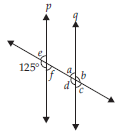SOLUTION: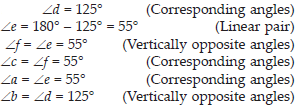Q 18.

Find the value of x in each of the following figures if l||m.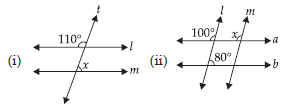SOLUTION: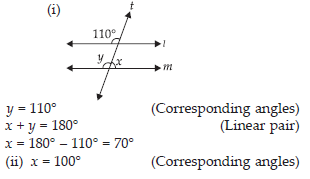Q 19.

In the given figure, the arms of two angles are parallel. If ∠ABC = 70°, then find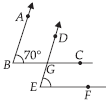(i) ∠DGC
(ii) ∠DEF

SOLUTION: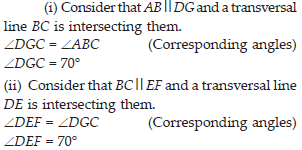Q 20.

In the given figures below, decide whether l is parallel to m.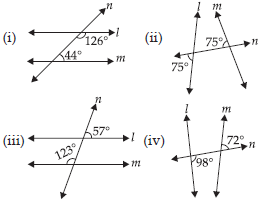SOLUTION:

(i) Consider two lines, l and m and a transversal line n which is intersecting them.
Sum of the interior angles on the same side of transversal = 126° + 44° = 170°. As the sum of interior angles on the same side of transversal is not 180°, therefore, l is not parallel to m.